Next: Analysis of the Up: The Model Previous: The Model

### Formulation

In the Simplified Global ... section we discussed various simplified versions of the overall model to be presented in this paper. There are some unanswered questions raised by these models, and in particular, that of the stability and flexibility of the system. In this section, we wish to present the more complete model and discuss in general how it functions. Furthermore, we will show how it possesses features able to give a broad range of responses to inputs. Most specifically, we wish to show that parameter ranges can be varied by internal and external factors.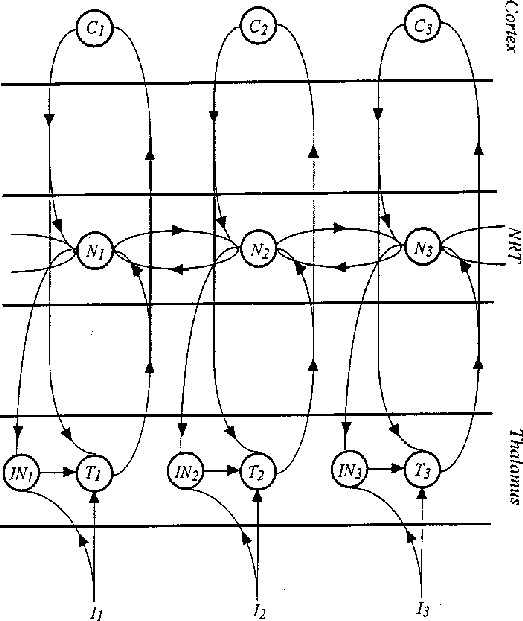Figure 2: The wiring diagram of the main model of the thalamus-NRT-cortex complex. Input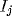is sent to both the thalamic relay cell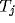and the inhibitory interneuron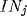, which itself feeds to. Output fromgoes up to the corresponding cortical cell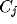, which feeds back its output to. Both the axons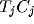and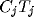send axon collaterals to the corresponding NRT cell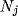. There is axonal output fromto, as well as collaterals to neighbouring NRT cells; there are also dendro-dendritic synapses between the NRT cells.

The basis of the model is best seen in terms of its wiring diagram in Figure 2. The model is an extension of that in Figure 1 by means of :

(i) addition of the cortical layer C,

(ii) addition of interneurons IN in thalamus,

(iii) extension of the input to IN cells as well as the T cells, and NRT feedback solely taken to IN cells.

The latter conectivity is an approximation to the results of the analysis of Steriade and colleagues , in which the effect of GABA on GABAergic cells (from N cell feedback to IN cell) is claimed to be an order of magnitude more effective than of GABA on excitatory cells. We note that the model may be regarded as the framework for more extensive modelling with layered cortex and NRT. However, at this stage, too much complexity would be introduced too rapidly by such structural richness.

We now turn to the detail of the equations used to describe the thalamus-NRT-cortex interacting system, whose general structure is shown in Figure 2. Excitatory neurons are assumed to be at a coordinate position(using the same coordinate frame for thalamus, NRT and cortex) on the thalamic and cortical sheets and to have membrane potentials labelled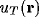and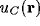, and outputs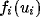, (i=T and C, respectively), where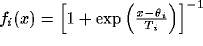, with threshold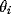and temperature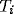. Inhibitory neurons in the thalamus and on the NRT (with the same coordinate positions) have membrane potentials denoted by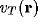,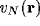, with similar sigmoidal outputs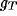,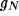to the other neurons. Connection weights from the j'th excitatory (inhibitory) neuron at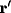to the i'th excitatory (inhibitory) neuron atare denoted by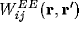,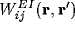,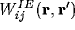,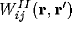. The resulting leaky integrator neurons (LINs) satisfy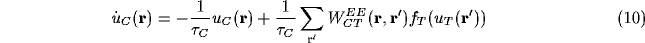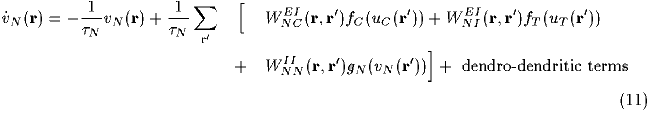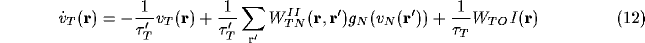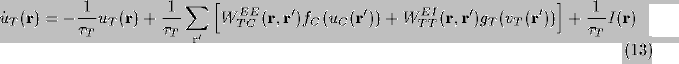(where we are using the notation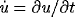). We have rescaled all the connection weights, and the input on the thalamic cells, so that the decay constants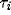,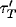drop out for stationary activity. Moreover the dendro-dendritic contribution has yet to be included in (11), it being given by the analysis of Taylor , as cited earlier, to be equal in the linearised limit to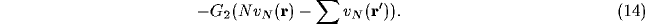A purely rectangular net with four neighbours at horizontal and vertical distances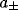,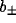(so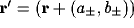) gives, in the continuum limit , the dendro-dendritic term is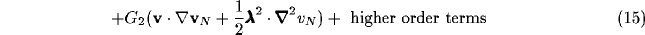where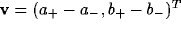,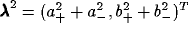,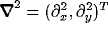, and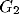is a negatively-valued constant in (15). The equation (11) thus reduces to a negative Laplacian net, with a linear derivative term proportional to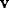. The inputs to the net depend, however, in a non-linear manner on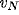as given by (12), (14), (15).Next: Analysis of the Up: The Model Previous: The Model